# RD Sharma solutions for Class 10 Maths chapter 2 - Polynomials [Latest edition]

#### Chapters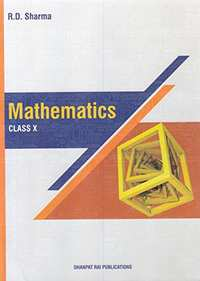## Chapter 2: Polynomials

Exercise 2.1Exercise 2.2Exercise 2.3Exercise 2.4Exercise 2.5
Exercise 2.1 [Pages 33 - 35]

### RD Sharma solutions for Class 10 Maths Chapter 2 Polynomials Exercise 2.1 [Pages 33 - 35]

Exercise 2.1 | Q 1.1 | Page 33

find the zeroes of the quadratic polynomial x2 – 2x – 8 and verify a relationship between zeroes and its coefficients

Exercise 2.1 | Q 1.2 | Page 33

Find the zeroes of the following quadratic polynomials and verify the relationship between the zeroes and the coefficients

4s2 – 4s + 1

Exercise 2.1 | Q 1.3 | Page 33

Find the zeroes of the following quadratic polynomials and verify the relationship between the zeroes and the coefficients

t2 – 15

Exercise 2.1 | Q 1.4 | Page 33

Find the zeroes of the following quadratic polynomials and verify the relationship between the zeroes and the coefficients

p(x) = x^2 + 2sqrt2x + 6

Exercise 2.1 | Q 1.5 | Page 33

Find the zeroes of the following quadratic polynomials and verify the relationship between the zeroes and the coefficients

q(x)=sqrt3x^2+10x+7sqrt3

Exercise 2.1 | Q 1.6 | Page 33

Find the zeroes of the following quadratic polynomials and verify the relationship between the zeroes and the coefficients

f(x)=x^2-(sqrt3+1)x+sqrt3

Exercise 2.1 | Q 1.7 | Page 33

Find the zeroes of the following quadratic polynomials and verify the relationship between the zeroes and the coefficients

g(x)=a(x^2+1)-x(a^2+1)

Exercise 2.1 | Q 1.8 | Page 33

Find the zeroes of the following quadratic polynomials and verify the relationship between the zeroes and the coefficients

6x^2-3-7x

Exercise 2.1 | Q 2.1 | Page 35

If α and β are the zeros of the quadratic polynomial f(x) = ax2 + bx + c, then evaluate α - β

Exercise 2.1 | Q 2.2 | Page 35

If α and β are the zeros of the quadratic polynomial f(x) = ax2 + bx + c, then evaluate 1/alpha-1/beta

Exercise 2.1 | Q 2.3 | Page 35

If α and β are the zeros of the quadratic polynomial f(x) = ax2 + bx + c, then evaluate 1/alpha+1/beta-2alphabeta

Exercise 2.1 | Q 2.4 | Page 35

If α and β are the zeros of the quadratic polynomial f(x) = ax2 + bx + c, then evaluate α2β + αβ2

Exercise 2.1 | Q 2.5 | Page 35

If α and β are the zeros of the quadratic polynomial f(x) = ax2 + bx + c, then evaluate α4 + β4

Exercise 2.1 | Q 2.6 | Page 35

If α and β are the zeros of the quadratic polynomial f(x) = ax2 + bx + c, then evaluate 1/(aalpha+b)+1/(abeta+b)

Exercise 2.1 | Q 2.7 | Page 35

If α and β are the zeros of the quadratic polynomial f(x) = ax2 + bx + c, then evaluate beta/(aalpha+b)+alpha/(abeta+b)

Exercise 2.1 | Q 2.8 | Page 35

If α and β are the zeros of the quadratic polynomial f(x) = ax2 + bx + c, then evaluate :

a(α^2/β+β^2/α)+b(α/β+β/α)

Exercise 2.1 | Q 3 | Page 34

If α and β are the zeros of the quadratic polynomial f(x) = 6x2 + x − 2, find the value of alpha/beta+beta/alpha

Exercise 2.1 | Q 4 | Page 34

If a and are the zeros of the quadratic polynomial f(x) = 𝑥2 − 𝑥 − 4, find the value of 1/alpha+1/beta-alphabeta

Exercise 2.1 | Q 5 | Page 34

If 𝛼 and 𝛽 are the zeros of the quadratic polynomial p(x) = 4x2 − 5x −1, find the value of α + αβ2.

Exercise 2.1 | Q 6 | Page 34

If a and 3 are the zeros of the quadratic polynomial f(x) = x2 + x − 2, find the value of 1/alpha-1/beta

Exercise 2.1 | Q 7 | Page 34

If 𝛼 and 𝛽 are the zeros of the quadratic polynomial f(x) = x2 − 5x + 4, find the value of 1/alpha+1/beta-2alphabeta

Exercise 2.1 | Q 8 | Page 34

If 𝛼 and 𝛽 are the zeros of the quadratic polynomial f(t) = t2 − 4t + 3, find the value of alpha^4beta^3+alpha^3beta^4

Exercise 2.1 | Q 9 | Page 34

If α and β are the zeros of the quadratic polynomial p(y) = 5y2 − 7y + 1, find the value of 1/alpha+1/beta

Exercise 2.1 | Q 10 | Page 34

If α and β are the zeros of the quadratic polynomial p(s) = 3s2 − 6s + 4, find the value of alpha/beta+beta/alpha+2[1/alpha+1/beta]+3alphabeta

Exercise 2.1 | Q 11 | Page 35

If α and β are the zeros of the quadratic polynomial f(x) = x2 − px + q, prove that alpha^2/beta^2+beta^2/alpha^2=p^4/q^2-(4p^2)/q+2

Exercise 2.1 | Q 12 | Page 35

If the squared difference of the zeros of the quadratic polynomial f(x) = x2 + px + 45 is equal to 144, find the value of p.

Exercise 2.1 | Q 13 | Page 34

If the sum of the zeros of the quadratic polynomial f(t) = kt2 + 2t + 3k is equal to their product, find the value of k.

Exercise 2.1 | Q 14 | Page 34

If one zero of the quadratic polynomial f(x) = 4x2 − 8kx − 9 is negative of the other, find the value of k.

Exercise 2.1 | Q 15 | Page 35

If α and β are the zeros of the quadratic polynomial f(x) = x2 − 1, find a quadratic polynomial whose zeroes are (2alpha)/beta" and "(2beta)/alpha

Exercise 2.1 | Q 16 | Page 35

If α and β are the zeros of the quadratic polynomial f(x) = x2 − 3x − 2, find a quadratic polynomial whose zeroes are 1/(2alpha+beta)+1/(2beta+alpha)

Exercise 2.1 | Q 17 | Page 35

If α and β are the zeros of a quadratic polynomial such that α + β = 24 and α − β = 8, find a quadratic polynomial having α and β as its zeros.

Exercise 2.1 | Q 18 | Page 35

If α and β are the zeros of the quadratic polynomial f(x) = x2 − p (x + 1) — c, show that (α + 1)(β +1) = 1− c.

Exercise 2.1 | Q 19.1 | Page 35

If If α and β are the zeros of the quadratic polynomial f(x) = x2 – 2x + 3, find a polynomial whose roots are α + 2, β + 2.

Exercise 2.1 | Q 19.2 | Page 35

If If α and β are the zeros of the quadratic polynomial f(x) = x2 – 2x + 3, find a polynomial whose roots are (alpha-1)/(alpha+1) , (beta-1)/(beta+1)

Exercise 2.1 | Q 20 | Page 35

If α and β are the zeroes of the polynomial f(x) = x2 + px + q, form a polynomial whose zeroes are (α + β)2 and (α − β)2.

Exercise 2.2 [Page 43]

### RD Sharma solutions for Class 10 Maths Chapter 2 Polynomials Exercise 2.2 [Page 43]

Exercise 2.2 | Q 1.1 | Page 43

Verify that the numbers given along side of the cubic polynomials are their zeroes. Also verify the relationship between the zeroes and the coefficients.

2x^3 + x^2 – 5x + 2 ; 1/2, 1, – 2

Exercise 2.2 | Q 1.2 | Page 43

Verify that the numbers given alongside of the cubic polynomials below are their zeroes. Also verify the relationship between the zeroes and the coefficients in each case

x3 – 4x2 + 5x – 2; 2, 1, 1

Exercise 2.2 | Q 2 | Page 43

Find a cubic polynomial with the sum, sum of the product of its zeroes taken two at a time, and product of its zeros as 3, −1 and −3 respectively.

Exercise 2.2 | Q 3 | Page 43

If the zeros of the polynomial f(x) = 2x3 − 15x2 + 37x − 30 are in A.P., find them.

Exercise 2.2 | Q 4 | Page 43

Find the condition that the zeros of the polynomial f(x) = x3 + 3px2 + 3qx + r may be in A.P.

Exercise 2.2 | Q 5 | Page 43

If the zeros of the polynomial f(x) = ax3 + 3bx2 + 3cx + d are in A.P., prove that 2b3 − 3abc + a2d = 0.

Exercise 2.2 | Q 6 | Page 43

If the zeros of the polynomial f(x) = x3 − 12x2 + 39x + k are in A.P., find the value of k.

Exercise 2.3 [Pages 57 - 58]

### RD Sharma solutions for Class 10 Maths Chapter 2 Polynomials Exercise 2.3 [Pages 57 - 58]

Exercise 2.3 | Q 1.1 | Page 57

Apply division algorithm to find the quotient q(x) and remainder r(x) on dividing f(x) by g(x) in the following f(x) = x3 − 6x2 + 11x − 6, g(x) = x2 + x + 1

Exercise 2.3 | Q 1.2 | Page 57

Apply division algorithm to find the quotient q(x) and remainder r(x) on dividing f(x) by g(x) in the following f(x) = 10x4 + 17x3 − 62x2 + 30x − 3, g(x) = 2x2 + 7x + 1

Exercise 2.3 | Q 1.3 | Page 57

Apply division algorithm to find the quotient q(x) and remainder r(x) on dividing f(x) by g(x) in the following f(x) = 4x3 + 8x2 + 8x + 7, g(x) = 2x2 − x + 1

Exercise 2.3 | Q 1.4 | Page 57

Apply division algorithm to find the quotient q(x) and remainder r(x) on dividing f(x) by g(x) in the following f(x) = 15x3 − 20x2 + 13x − 12; g(x) = x2 − 2x + 2

Exercise 2.3 | Q 2.1 | Page 57

Check whether the first polynomial is a factor of the second polynomial by dividing the second polynomial by the first polynomial

t2 – 3, 2t4 + 3t3 – 2t2 – 9t – 12

Exercise 2.3 | Q 2.2 | Page 57

Check whether the first polynomial is a factor of the second polynomial by dividing the second polynomial by the first polynomial

x3 – 3x + 1, x5 – 4x3 + x2 + 3x + 1

Exercise 2.3 | Q 2.3 | Page 57

Check whether the first polynomial is a factor of the second polynomial by applying the division algorithm g(x) = 2x2 − x + 3, f(x) = 6x5 − x4 + 4x3 − 5x2 − x − 15

Exercise 2.3 | Q 4 | Page 57

Obtain all zeros of f(x) = x3 + 13x2 + 32x + 20, if one of its zeros is −2.

Exercise 2.3 | Q 5 | Page 57

Obtain all zeros of the polynomial f(x) = x4 − 3x3 − x2 + 9x − 6, if two of its zeros are -sqrt3 and sqrt3

Exercise 2.3 | Q 6 | Page 57

Find all zeros of the polynomial f(x) = 2x4 − 2x3 − 7x2 + 3x + 6, if its two zeros are -sqrt(3/2) and sqrt(3/2)

Exercise 2.3 | Q 7 | Page 58

What must be added to the polynomial f(x) = x4 + 2x3 − 2x2 + x − 1 so that the resulting polynomial is exactly divisible by x2 + 2x − 3 ?

Exercise 2.3 | Q 8 | Page 58

What must be subtracted from the polynomial f(x) = x4 + 2x3 − 13x2 − 12x + 21 so that the resulting polynomial is exactly divisible by x2 − 4x + 3 ?

Exercise 2.3 | Q 9 | Page 57

Find all the zeros of the polynomial x4 + x3 − 34x2 − 4x + 120, if two of its zeros are 2 and −2.

Exercise 2.3 | Q 10 | Page 57

Find all zeros of the polynomial 2x4 + 7x3 − 19x2 − 14x + 30, if two of its zeros are sqrt2 and -sqrt2.

Exercise 2.3 | Q 11 | Page 58

Find all the zeros of the polynomial 2x3 + x2 − 6x − 3, if two of its zeros are -sqrt3 and sqrt3

Exercise 2.3 | Q 12 | Page 58

Find all the zeros of the polynomial x3 + 3x2 − 2x − 6, if two of its zeros are -sqrt2 and sqrt2

Exercise 2.4 [Pages 58 - 61]

### RD Sharma solutions for Class 10 Maths Chapter 2 Polynomials Exercise 2.4 [Pages 58 - 61]

Exercise 2.4 | Q 1 | Page 58

Define a polynomial with real coefficients.

Exercise 2.4 | Q 2 | Page 58

Define degree of a polynomial.

Exercise 2.4 | Q 3 | Page 58

Write the standard form of a linear polynomial with real coefficients.

Exercise 2.4 | Q 4 | Page 58

Write the standard form of a quadratic polynomial with real coefficients.

Exercise 2.4 | Q 5 | Page 58

Write the standard form of a cubic polynomial with real coefficients.

Exercise 2.4 | Q 6 | Page 58

Define value of polynomial at a point.

Exercise 2.4 | Q 7 | Page 58

Define the zero of a polynomial.

Exercise 2.4 | Q 8 | Page 58

The sum and product of the zeros of a quadratic polynomial are $- \frac{1}{2}$ and −3 respectively. What is the quadratic polynomial.

Exercise 2.4 | Q 9 | Page 58

Write the family of quadratic polynomials having $- \frac{1}{4}$ and 1 as its zeros.

Exercise 2.4 | Q 10 | Page 58

If the product of zeros of the quadratic polynomial f(x) = x2 − 4x + k is 3, find the value of k.

Exercise 2.4 | Q 11 | Page 58

If the sum of the zeros of the quadratic polynomial f(x) = kx2 − 3x + 5 is 1, write the value of k.

Exercise 2.4 | Q 12 | Page 59

In Fig. 2.17, the graph of a polynomial p(x) is given. Find the zeros of the polynomial.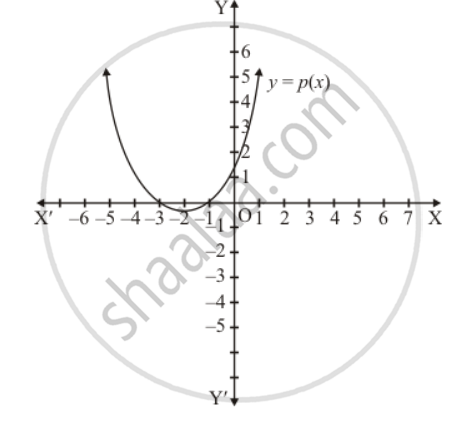Exercise 2.4 | Q 13 | Page 59

The graph of a polynomial y = f(x), shown in Fig. 2.18. Find the number of real zeros of f(x).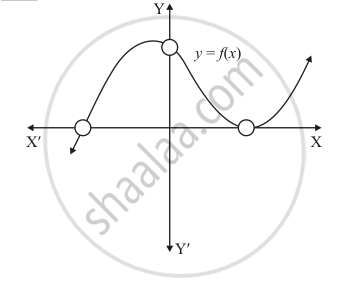Exercise 2.4 | Q 14 | Page 59

The graph of the polynomial f(x) = ax2 + bx + c is as shown below (Fig. 2.19). Write the signs of 'a' and b2 − 4ac.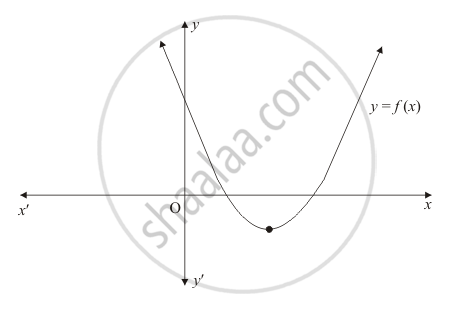Exercise 2.4 | Q 15 | Page 59

The graph of the polynomial f(x) = ax2 + bx + c is as shown in Fig. 2.20. Write the value of b2 − 4ac and the number of real zeros of f(x).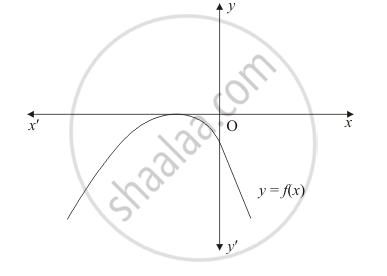Exercise 2.4 | Q 16 | Page 59

In Q. No. 14, write the sign of c.

Exercise 2.4 | Q 17 | Page 59

In Q. No. 15, write the sign of c.

Exercise 2.4 | Q 18 | Page 59

The graph of a polynomial f(x) is as shown in Fig. 2.21. Write the number of real zeros of f(x).Exercise 2.4 | Q 19 | Page 59

If x = 1 is a zero of the polynomial f(x) = x3 − 2x2 + 4x + k, write the value of k.

Exercise 2.4 | Q 20 | Page 59

State division algorithm for polynomials.

Exercise 2.4 | Q 21 | Page 59

Give an example of polynomials f(x), g(x), q(x) and r(x) satisfying f(x) = g(x), q(x) + r(x), where degree r(x) = 0.

Exercise 2.4 | Q 22 | Page 60

Write a quadratic polynomial, sum of whose zeros is $2\sqrt{3}$ and their product is 2.

Exercise 2.4 | Q 23 | Page 60

If fourth degree polynomial is divided by a quadratic polynomial, write the degree of the remainder.

Exercise 2.4 | Q 24 | Page 60

If f(x) = x3 + x2 − ax + b is divisible by x2 − x write the value of a and b.

Exercise 2.4 | Q 25 | Page 60

If a − ba and b are zeros of the polynomial f(x) = 2x3 − 6x2 + 5x − 7, write the value of a.

Exercise 2.4 | Q 26 | Page 60

Write the coefficient of the polynomial p(z) = z5 − 2z2 + 4.

Exercise 2.4 | Q 27 | Page 60

Write the zeros of the polynomial x2 − x − 6.

Exercise 2.4 | Q 28 | Page 60

If (x + a) is a factor of 2x2 + 2ax + 5x + 10, find a.

Exercise 2.4 | Q 29 | Page 60

For what value of k, −4 is a zero of the polynomial x2 − x − (2k + 2)?

Exercise 2.4 | Q 30 | Page 60

If 1 is a zero of the polynomial p(x) = ax2 − 3(a − 1) x − 1, then find the value of a.

Exercise 2.4 | Q 31 | Page 60

If α, β are the zeros of a polynomial such that α + β = −6 and αβ = −4, then write the polynomial.

Exercise 2.4 | Q 32 | Page 60

If α, β are the zeros of the polynomial 2y2 + 7y + 5, write the value of α + β + αβ.

Exercise 2.4 | Q 33 | Page 60

For what value of k, is 3 a zero of the polynomial 2x2 + x + k?

Exercise 2.4 | Q 34 | Page 60

For what value of k, is −3 a zero of the polynomial x2 + 11x + k?

Exercise 2.4 | Q 35 | Page 60

For what value of k, is −2 a zero of the polynomial 3x2 + 4x + 2k?

Exercise 2.4 | Q 36 | Page 60

If a quadratic polynomial f(x) is factorizable into linear distinct factors, then what is the total number of real and distinct zeros of f(x)?

Exercise 2.4 | Q 37 | Page 61

If a quadratic polynomial f(x) is a square of a linear polynomial, then its two zeros are coincident. (True/False).

Exercise 2.4 | Q 38 | Page 61

If a quadratic polynomial f(x) is not factorizable into linear factors, then it has no real zero. (True/False)

Exercise 2.4 | Q 39 | Page 61

If f(x) is a polynomial such that f(af(b) < 0, then what is the number of zeros lying between a and b?

Exercise 2.4 | Q 40 | Page 61

If graph of quadratic polynomial ax2 + bx + c cuts positive direction of y-axis, then what is the sign of c?

Exercise 2.4 | Q 41 | Page 61

If the graph of quadratic polynomial ax2 + bx + c cuts negative direction of y-axis, then what is the sign of c?

Exercise 2.5 [Pages 61 - 64]

### RD Sharma solutions for Class 10 Maths Chapter 2 Polynomials Exercise 2.5 [Pages 61 - 64]

Exercise 2.5 | Q 1 | Page 61

If α, β are the zeros of the polynomial f(x) = x2 + x + 1, then $\frac{1}{\alpha} + \frac{1}{\beta} =$

• 1

• -1

• 0

• None of these

Exercise 2.5 | Q 2 | Page 61

If α, β are the zeros of the polynomial p(x) = 4x2 + 3x + 7, then $\frac{1}{\alpha} + \frac{1}{\beta}$  is equal to

• $\frac{7}{3}$
• $- \frac{7}{3}$
• $\frac{3}{7}$
• $- \frac{3}{7}$
Exercise 2.5 | Q 3 | Page 61

If one zero of the polynomial f(x) = (k2 + 4)x2 + 13x + 4k is reciprocal of the other, then k=

• 2

• -2

• 1

• -1

Exercise 2.5 | Q 4 | Page 62

If the sum of the zeros of the polynomial f(x) = 2x3 − 3kx2 + 4x − 5 is 6, then the value ofk is

• 2

• 4

•  −2

• −4

Exercise 2.5 | Q 5 | Page 61

If α and β are the zeros of the polynomial f(x) = x2 + px + q, then a polynomial having $\frac{1}{\alpha} \text{and}\frac{1}{\beta}$  is its zero is

•  x2 + qx + p

• x2 − px + q

• qx2 + px + 1

• px2 + qx + 1

Exercise 2.5 | Q 6 | Page 61

If α, β are the zeros of polynomial f(x) = x2 − p (x + 1) − c, then (α + 1) (β + 1) =

• c − 1

• 1 − c

• c

• 1 + c

Exercise 2.5 | Q 7 | Page 61

If α, β are the zeros of the polynomial f(x) = x2 − p(x + 1) − c such that (α +1) (β + 1) = 0, then c =

• 1

• 0

• -1

• 2

Exercise 2.5 | Q 8 | Page 61

If f(x) = ax2 + bx + c has no real zeros and a + b + c = 0, then

• c = 0

• c > 0

• < 0

• None of these

Exercise 2.5 | Q 9 | Page 61

If the diagram in Fig. 2.22 shows the graph of the polynomial f(x) = ax2 + bx + c, then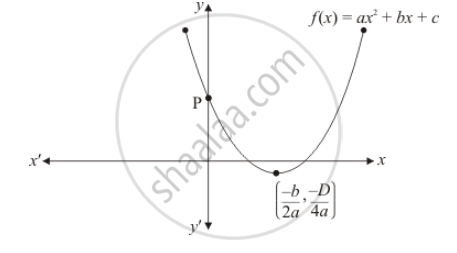• a > 0, b < 0 and c > 0

• a < 0, b < 0 and c < 0

• a < 0, b > 0 and c > 0

• a < 0, b > 0 and c < 0

Exercise 2.5 | Q 10 | Page 61

Figure 2.23 show the graph of the polynomial f(x) = ax2 + bx + c for which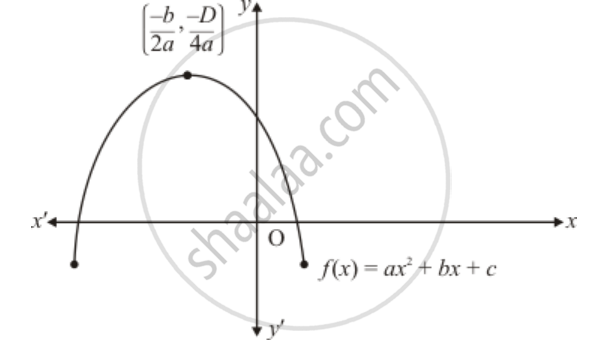• a < 0, b > 0 and c > 0

• a < 0, b < 0 and c > 0

• a < 0, b < 0 and c < 0

• a > 0, b > 0 and c < 0

Exercise 2.5 | Q 11 | Page 62

If the product of zeros of the polynomial f(xax3 − 6x2 + 11x − 6 is 4, then a =

• $\frac{3}{2}$

• $- \frac{3}{2}$

• $\frac{2}{3}$

• $- \frac{2}{3}$

• $- \frac{2}{3}$

Exercise 2.5 | Q 12 | Page 62

If zeros of the polynomial f(x) = x3 − 3px2 + qx − r are in A.P., then

• 2p3 = pq − r

• 2p3 = pq + r

•  p3 = pq − r

• None of these

Exercise 2.5 | Q 13 | Page 63

If the product of two zeros of the polynomial f(x) = 2x3 + 6x2 − 4x + 9 is 3, then its third zero is

• $\frac{3}{2}$

• $- \frac{3}{2}$
• $\frac{9}{2}$
• $- \frac{9}{2}$
Exercise 2.5 | Q 14 | Page 63

If the polynomial f(x) = ax3 + bx − c is divisible  by the polynomial g(x) = x2 + bx + c, then ab =

•  1

• $\frac{1}{c}$
• −1

• $- \frac{1}{c}$
Exercise 2.5 | Q 15 | Page 63

If Q.No. 14, c =

•  b

• 2b

• 2b2

• −2b

Exercise 2.5 | Q 16 | Page 63

If one root of the polynomial f(x) = 5x2 + 13x + k is reciprocal of the other, then the value of k is

• 0

• 5

• $\frac{1}{6}$
• 6

Exercise 2.5 | Q 17 | Page 63

If α, β, γ are the zeros of the polynomial f(x) = ax3 + bx2 + cx + d, the$\frac{1}{\alpha} + \frac{1}{\beta} + \frac{1}{\gamma} =$

• $- \frac{b}{d}$
• $\frac{c}{d}$
• $- \frac{c}{d}$
• $- \frac{c}{a}$
Exercise 2.5 | Q 18 | Page 63

If α, β, γ are the zeros of the polynomial f(x) = ax3 + bx2 cx + d, then α2 + β2 + γ2 =

• $\frac{b^2 - ac}{a^2}$
• $\frac{b^2 - 2ac}{a}$
• $\frac{b^2 + 2ac}{b^2}$
• $\frac{b^2 - 2ac}{a^2}$
Exercise 2.5 | Q 19 | Page 63

If α, β, γ are are the zeros of the polynomial f(x) = x3 − px2 + qx − r, the$\frac{1}{\alpha\beta} + \frac{1}{\beta\gamma} + \frac{1}{\gamma\alpha} =$

Exercise 2.5 | Q 20 | Page 63

If α, β are the zeros of the polynomial f(x) = ax2 + bx + c, then$\frac{1}{\alpha^2} + \frac{1}{\beta^2} =$

• $\frac{b^2 - 2ac}{a^2}$
• $\frac{b^2 - 2ac}{c^2}$
• $\frac{b^2 + 2ac}{a^2}$
• $\frac{b^2 + 2ac}{c^2}$
Exercise 2.5 | Q 21 | Page 63

If two of the zeros of the cubic polynomial ax3 + bx2 + cx + d are each equal to zero, then the third zero is

• $\frac{- d}{a}$
• $\frac{c}{a}$
• $\frac{- b}{a}$
• $\frac{b}{a}$
Exercise 2.5 | Q 22 | Page 63

If two zeros x3 + x2 − 5x − 5 are $\sqrt{5}\ \text{and} - \sqrt{5}$, then its third zero is

•  1

• −1

• 2

• −2

Exercise 2.5 | Q 23 | Page 63

The product of the zeros of x3 + 4x2 + x − 6 is

• −4

• 4

• 6

• −6

Exercise 2.5 | Q 24 | Page 64

What should be added to the polynomial x2 − 5x + 4, so that 3 is the zero of the resulting polynomial?

• 1

• 2

• 4

• 5

Exercise 2.5 | Q 25 | Page 64

What should be subtracted to the polynomial x2 − 16x + 30, so that 15 is the zero of the resulting polynomial?

• 30

• 14

• 15

• 16

Exercise 2.5 | Q 26 | Page 64

A quadratic polynomial, the sum of whose zeroes is 0 and one zero is 3, is

• x2 − 9

• x2 + 9

• x2 + 3

• x2 − 3

Exercise 2.5 | Q 27 | Page 64

If two zeroes of the polynomial x3 + x2 − 9x − 9 are 3 and −3, then its third zero is

• -1

• 1

• -9

• 9

Exercise 2.5 | Q 28 | Page 64

If $\sqrt{5}\ \text{and} - \sqrt{5}$   are two zeroes of the polynomial x3 + 3x2 − 5x − 15, then its third zero is

•  3

• -3

• 5

• -5

Exercise 2.5 | Q 29 | Page 63

If x + 2 is a factor of x2 + ax + 2b and a + b = 4, then

• a= 1, b = 3

• a = 3, b = 1

• a = −1, b = 5

• a = 5, b = −1

Exercise 2.5 | Q 30 | Page 64

The polynomial which when divided by −x2 + x − 1 gives a quotient x − 2 and remainder 3, is

• x3 − 3x2 + 3x − 5

• x3 − 3x2 − 3x − 5

• x3 + 3x2 − 3x + 5

• x3 − 3x2 − 3x + 5

## Chapter 2: Polynomials

Exercise 2.1Exercise 2.2Exercise 2.3Exercise 2.4Exercise 2.5## RD Sharma solutions for Class 10 Maths chapter 2 - Polynomials

RD Sharma solutions for Class 10 Maths chapter 2 (Polynomials) include all questions with solution and detail explanation. This will clear students doubts about any question and improve application skills while preparing for board exams. The detailed, step-by-step solutions will help you understand the concepts better and clear your confusions, if any. Shaalaa.com has the CBSE Class 10 Maths solutions in a manner that help students grasp basic concepts better and faster.

Further, we at Shaalaa.com provide such solutions so that students can prepare for written exams. RD Sharma textbook solutions can be a core help for self-study and acts as a perfect self-help guidance for students.

Concepts covered in Class 10 Maths chapter 2 Polynomials are Geometrical Meaning of the Zeroes of a Polynomial, Relationship Between Zeroes and Coefficients of a Polynomial, Division Algorithm for Polynomials, Polynomials, Polynomials.

Using RD Sharma Class 10 solutions Polynomials exercise by students are an easy way to prepare for the exams, as they involve solutions arranged chapter-wise also page wise. The questions involved in RD Sharma Solutions are important questions that can be asked in the final exam. Maximum students of CBSE Class 10 prefer RD Sharma Textbook Solutions to score more in exam.

Get the free view of chapter 2 Polynomials Class 10 extra questions for Class 10 Maths and can use Shaalaa.com to keep it handy for your exam preparation